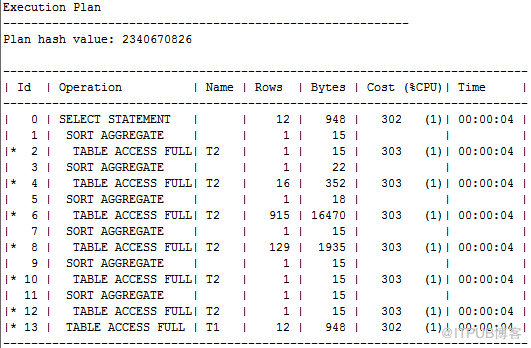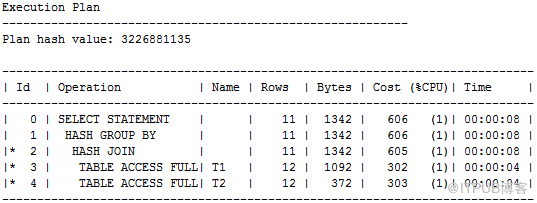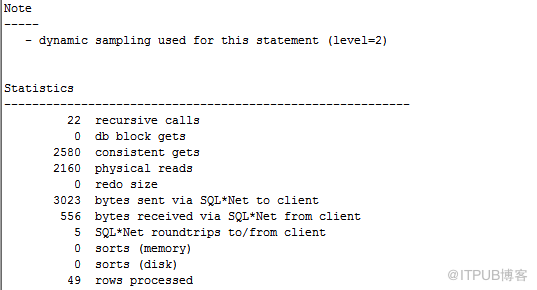--- 说明：案例来自《 收获，不止SQL 优化

SQL > drop table t1 purge ;
SQL > drop table t2 purge ;
SQL > create table t1 as select * from dba_objects ;
SQL > create table t2 as select * from dba_objects ;
SQL > update t2 set status = 'INVALID' WHERE ROWNUM <= 10000 ;
SQL > update t2 set generated = 'Y' WHERE ROWNUM <= 10000 ;
SQL > update t2 set temporary = 'Y' WHERE ROWNUM <= 10000 ;
SQL > update t2 set temporary = 'M' WHERE temporary <> 'Y' ;
SQL > update t2 set temporary = 'Q' WHERE temporary <> 'Y' or temporary <> 'M' ;
SQL > COMMIT ;
SQL > set autotrace traceonly
SQL > set linesize 1000
SQL ：

SQL>
select t1.object_name,
t1.object_id,
(select count(*)
from t2
where temporary = 'Y'
and t2.object_id = t1.object_id) CNT_TEMPORARY_Y,
(select count(*)
from t2
where created >= sysdate - 365
and t2.object_id = t1.object_id) CNT_CREATED_NEW,
(select sum(object_id)
from t2
where status <> 'VALUD'
and t2.object_id = t1.object_id) SUM_OBJID_STATUS_V,
(select sum(object_id)
from t2
where generated = 'Y'
and t2.object_id = t1.object_id) SUM_OBJID_GENERATED_Y,
(select sum(object_id)
from t2
where generated = 'M'
and t2.object_id = t1.object_id) SUM_OBJID_GENERATED_M,
(select sum(object_id)
from t2
where generated = 'Q'
and t2.object_id = t1.object_id) SUM_OBJID_GENERATED_Q
from t1
where t1.object_id <= 50;case when改造 后的 SQL

with w_t2 as
(select
t2.object_id,
count(case when t2.temporary='Y' then 1 end ) CNT_TEMPORARY_Y,
count(case when created >=sysdate-365  then 1 end ) CNT_CREATED_NEW,
sum(case when t2.status<>'VALID' then t2.object_id end ) SUM_OBJID_STATUS_V,
sum(case when t2.generated = 'Y' then t2.object_id end ) SUM_OBJID_GENERATED_Y,
sum(case when t2.generated = 'M' then t2.object_id end ) SUM_OBJID_GENERATED_M,
sum(case when t2.generated = 'Q' then t2.object_id end ) SUM_OBJID_GENERATED_Q
from  t2
group by t2.object_id)
select t1.object_name,t1.object_id,w_t2.* from t1,w_t2
where t1.object_id=w_t2.object_id
and t1.object_id<=50;